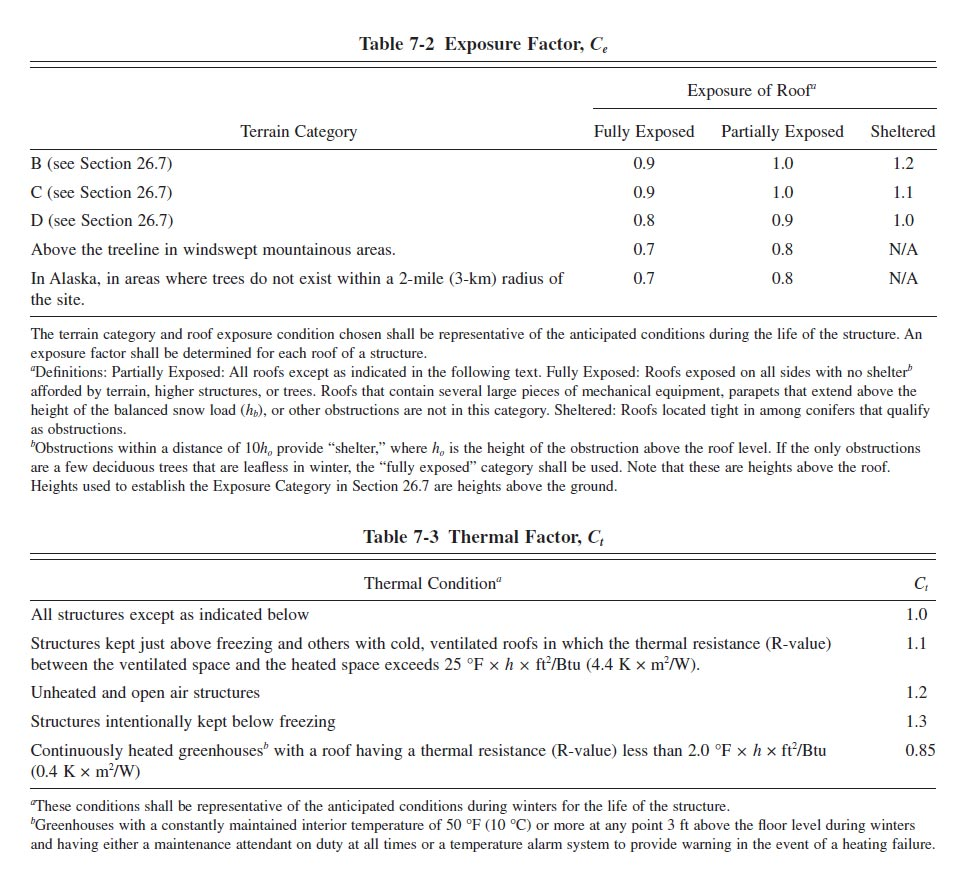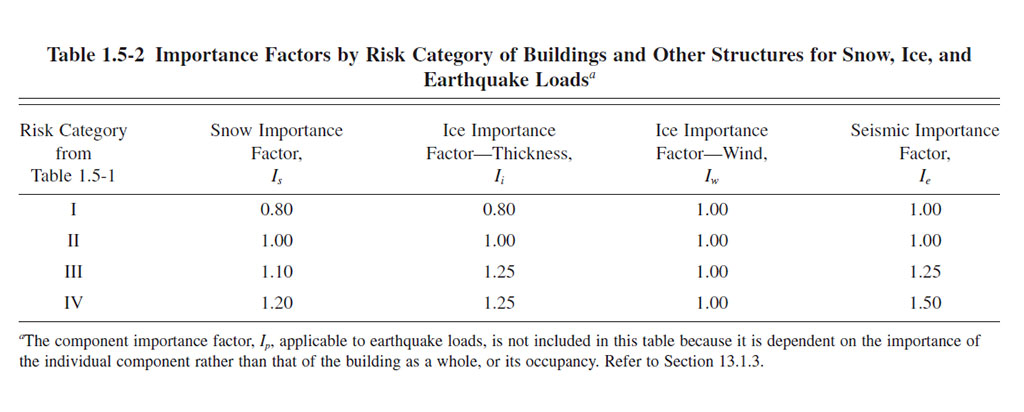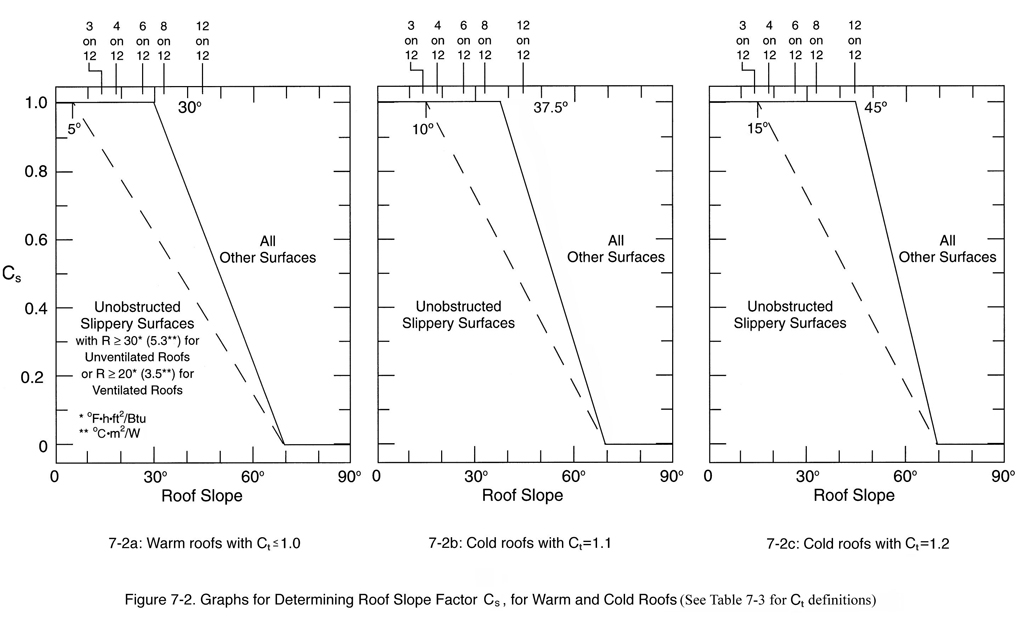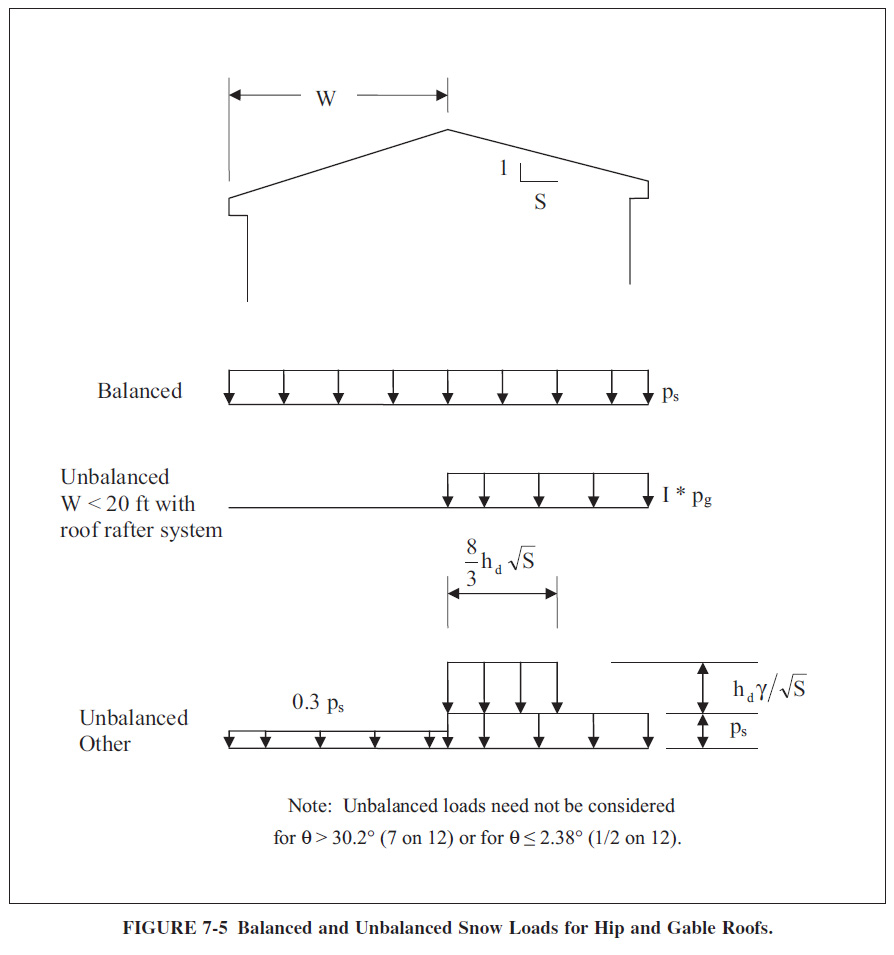Roof snow loads are influenced by elevation, general weather and moisture patterns, slope direction, exposure, roof configuration and wind direction and severity. Overestimation of snow loads can unnecessarily increase the cost of construction. Underestimation of snow loads can result in premature failure, and in some cases hazardous safety issues.

Most building codes in the United States reference the procedures found in ASCE 7 - Chapter 7 (Snow Loads). Roof snow loads are based on the historical records for ground snow loads for a given location, however the local building authority should always be consulted to determine the correct roof snow load to use for a given site and application.

The ground snow load map shown below was scanned from the ASCE 7-10 (Figure 7-1). Ground snow loads, pg, for the contiguous United States can generally be determined using this map. Ground snow loads for sites at elevations above the limits indicated and for all sites labeled "CS" require site-specific case studies and approval from the governing building authority. Ground snow loads for Alaska are given by Table 7.1 from the ASCE 7-10. Ground snow loads for Hawaii are zero, except in mountainous regions as determined by the authority having jurisdiction.

Please feel free to use our interactive ASCE Ground Snow Loads map for the contiguous United States.

If you need to gather ground snow load data programmatically, please consider our API Service.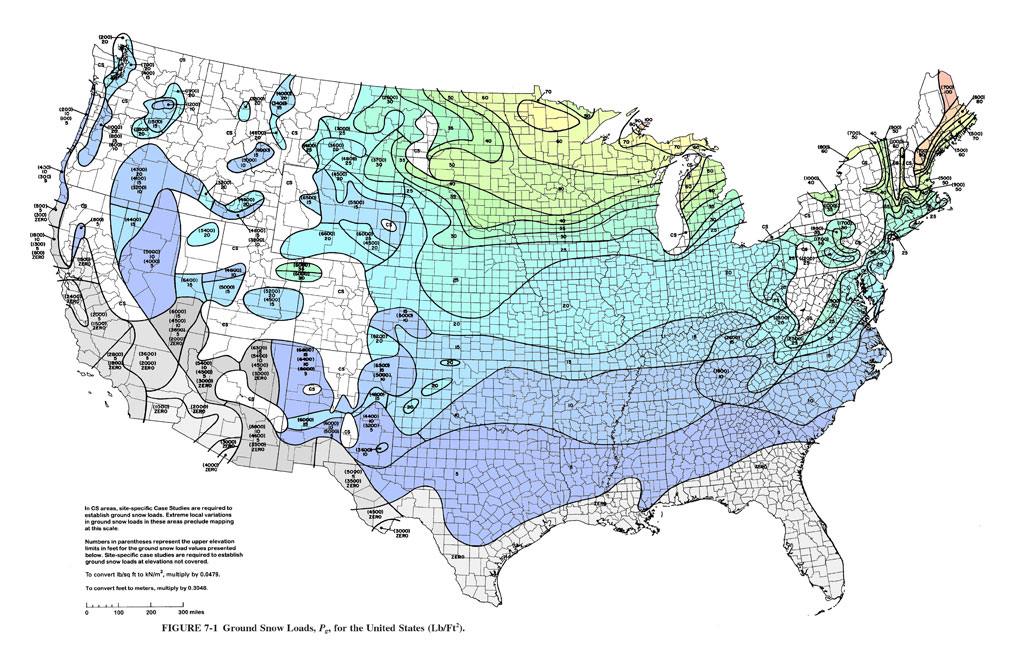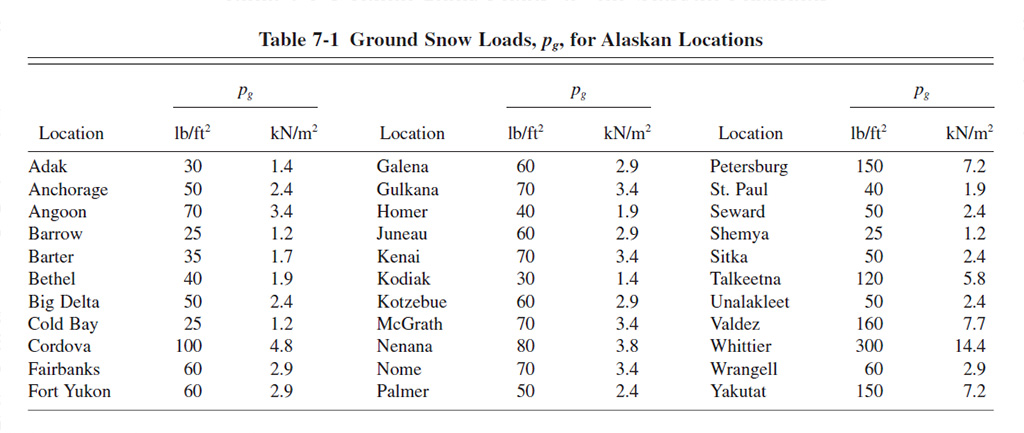Snow load calculations for most residential structures are usually performed using the following method:

• Determine ground snow load based on location and elevation
• Calculate flat roof snow load pf using the following equation: pf = 0.7CeCtIspg
where:
pf = Flat Roof Snow Load in psf
Ce = Exposure Factor, as determined by ASCE Table 7-2 below.
Most residential structures are situated in a B or C terrain category and are partially exposed with a Ce=1.0
Ct = Thermal Factor, as determined by ASCE Table 7-3 below.
Most modern residential structures have cold ventilated roofs with Ct=1.1
Is = Importance Factor, as determined by ASCE Table 1.5-2 below.
Most residential structures are Risk Category II with an Is=1.0
pg = Ground Snow Load in psf
• Check low sloped roofs for minimum snow load as per ASCE Sec. 7.3.4
• Where pg is less than or equal to 20 psf and slope is less than W/50 apply an additional 5 psf rain-on-snow surcharge load, as per ASCE Sec. 7.10
• Calculate sloped roof snow load ps using the following equation: ps = Cspf
where:
ps = Sloped Roof Snow Load in psf
Cs = Roof Slope Factor, as determined by ASCE Sec. 7.4.1-7.4.4 and ASCE Figure 7-2 below.
Residential roof with asphalt, wood shingles or shakes would use Fig. 7-2b with a Cs=1.0
pf = Flat Roof Snow Load in psf
• Calculate unbalanced snow load for hip and gable roofs as shown in ASCE Figure 7-5 below.
Unbalanced snow loads are required for roof pitches between 1/2 on 12 to 7 on 12.
Using the following equations:

γ = 0.13pg + 14 (snow density)
$${h_d} = \style{font-family:verdana}{.43}\sqrt[\style{font-family:verdana}{3}]{{{l_u}}} \sqrt[\style{font-family:verdana}{4}]{{{p_g} + \style{font-family:verdana}{10}}} - \style{font-family:verdana}{1.5}$$ (drift height) [if lu < 20 ft., use lu = 20 ft.]
$$l_d = \frac{\style{font-family:verdana}{8}}{\style{font-family:verdana}{3}} h_d \sqrt{S}$$ (width of drift surcharge)
$${p_d} = {h_d}\gamma /\sqrt S$$ (drift surcharge snow load)

where:
γ = Snow density in pcf, not to exceed 30 pcf.
hd = Drift height in feet, as determined by equation or ASCE Figure 7-9.
lu = W = Ridge to eave distance in feet, for windward portion of roof.
S = 12/Roof Pitch
ld = Width of drift surcharge in feet.
pd = Drift Surcharge Snow Load in psf
• On warm roofs apply a distributed 2pf snow load on all overhanging portions.